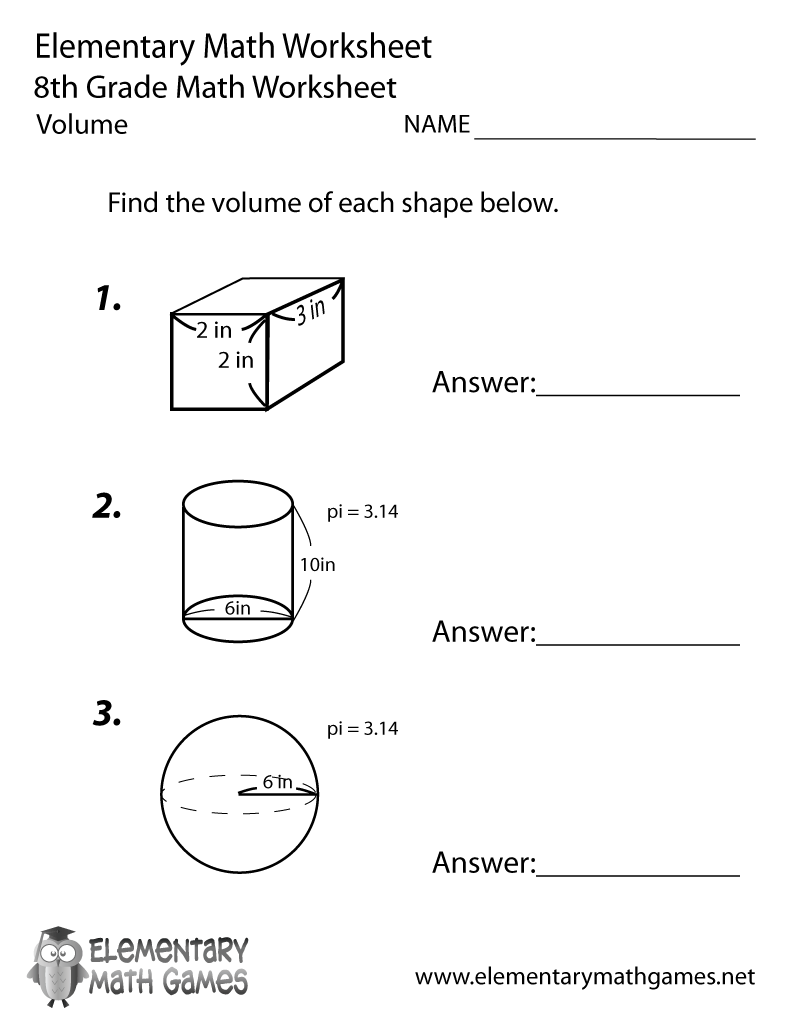# Eighth Grade Volume Worksheet## Learn and Practice Finding the Volume

This eighth grade math worksheet is a great way for students to practice volume. Eighth graders have to find the volume of different shapes.

Here are the two versions of this free eighth grade math worksheet: